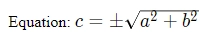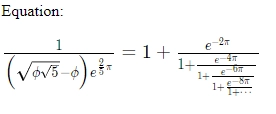# Introducing KaTeX (Math) and Embed Support

We are excited to introduce KaTeX and embedding support for comments in Hyvor Talk.

We are excited to introduce KaTeX and embedding support for comments in Hyvor Talk.

## KaTeX

KaTeX is the fastest library for math typesetting developed by Khan Academy. It uses Knuth's TeX format. Therefore, you can write math in comments in the same way you would in MathJax or other libraries.

### Enabling KaTeX

KaTeX support for comments is disabled by default. If your audience prefers writing comments with math, you can enable it from the console.

You can add inline math notations easily by adding valid KaTeX syntax between $$ tags. Ex: 1Equation:$$ c = \pm\sqrt{a^2 + b^2} $$ will be converted to#### 2. Block KaTeX (Multiline) If you want to add KaTeX as a block element, you can do it as follows. 1KaTex Example23$$4\frac{1}{\Bigl(\sqrt{\phi \sqrt{5}}-\phi\Bigr) e^{\frac25 \pi}} = 1+\frac{e^{-2\pi}} {1+\frac{e^{-4\pi}} {1+\frac{e^{-6\pi}} {1+\frac{e^{-8\pi}} {1+\cdots} } } }5$$ This will be converted to:• Start block KaTeX with $$ in one line

• End the block with .

## Embeds

Hyvor Talk will automatically detect embeddable links and convert them to an iframe or another embed format. All you have to do is to add the link in a new line.

1Creepy Mr.Bean23https://www.youtube.com/watch?v=l7A6jNOewFA

These embeds are currently supported

• Vimeo videos

• Giphy GIFs (No need to add them as images in markdown)

• Instagram posts